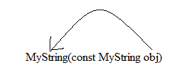# Copy Constructor in C++

## What is Copy Constructor?

Copy constructor creates a new object of the same class using an existing object. It creates a copy/replica of the existing object.

### Syntax of Copy Constructor

`( const  &givenObject)`

Here givenObject is the object of same class which is already created. Copy constructor takes its own class object (which already exists) as parameter while constructing another object.

## What Copy Constructor does?

It copies value of all member variables from existing object to new object. It copies values member-to-member.

### Example of Copy Constructor

```#include <string.h>
#include <iostream>
using namespace std;

class Point
{

public:
Point()//default constructor
{
cout << "defalut constructor is called to construct the object" << endl;
};

~Point(){};

Point(const int &xValue, const int &yValue)  //parameterized constructor
{
x = xValue;
y = yValue;
cout << "parameterized constructor is called to construct the object" << endl;
}

Point(const Point& pointObj) //copy constructor
{
x = pointObj.x;
y = pointObj.y;
cout << "copy constructor is called to construct the object" << endl;
}

void Print()
{
cout << "X-cordinate of point is : " << x << endl;
cout << "Y-cordinate of point is : " << y << endl;
}

private:
int x;
int y;
};

int main()
{
Point p1;  //default constructor is called to constrct object 'p1'

Point p2(10, 20);   //parameterized constructor is called to construct object 'p2'

Point cp(p2); //copy constructor is called to construct object 'cp'

cout << "print p1" << endl;

p1.Print();

cout << "print p2" << endl;

p2.Print();

cout << "print xp" << endl;

cp.Print();

return 0;
}```

### When is copy constructor called?

1. When an object is constructed based on another object of the same class.
2. When an object of the class is passed (to a function) by value as an argument.
3. When an object of the class is returned by value.
4. When compiler generates a temporary object.

## When you need a copy constructor?

If the class has pointer variables and has some dynamic memory allocations, then it is a must to have a copy constructor.

## What if you don’t define a copy constructor for a class?

If you don’t define copy constructor, the C++ compiler creates a default copy constructor for each class which does a member-wise copy (shallow copy) between objects.

## Then why we need a copy constructor?

Since copy constructor does a member-wise copy and if the class has some pointer member variable where memory allocation/deallocation is done specifically while constructing a new object a new memory block need to be allocated corresponding pointer member variable.

Example: In class string we must need to define a constructor as it has a pointer *name and we are allocating memory dynamically to it using `new`.

```#include
#include

using namespace std;

class MyString
{

private:

char *name;
int size;

public:

~MyString() { delete[] name; } // destructor

MyString(const char *str = NULL) // constructor
{
size = strlen(str);
name = new char[size + 1];
//strcpy(dst, src);
strcpy(name, str);
}

MyString(const MyString& old_str) // copy constructor
{
size = old_str.size;
name = new char[size + 1];
strcpy(name, old_str.name);
}

void Print()
{
cout << "name:" << name << endl;
}

};

int main()
{
//Example 1:
MyString str1("Hi");

str1.Print();

MyString str2(str1); //copy constructor called

str2.Print(); //This will crash if copy constructor not handled appropriately

//Example:2
MyString *str3 = new MyString("Hello");

if (str3)
{
str3->Print();
MyString str4(*str3);
delete str3;
str4.Print();
}
return 0;
}```

Comment copy constructor and then try to example 1 and example 2, one by one. str2.Print() and str4.Print() – will crash as there is no dynamic allocation done while creating str2 and str4.

## Difference between Default Constructor and Copy Constructor

 Default Constructor Copy Constructor Syntax () ( const &givenObject) Usage Create a new object Creates a new object from an existing object and does member-wise copy Parameter Doesn’t take any argument It takes its own class object as a parameter What if user doesn’t provide Compiler provides it Compiler provides it, but default copy constructor doesn’t do dynamic allocation

## Difference between Parameterized Constructor and Copy Constructor

Copy constructor’s syntax is fixed it is always defined as – It always excepts an existing object of same class as “const reference

### Syntax

Copy Constructor

`( const &givenObject)`

Parameterized constructor can be defined to accepts any type of arguments

`Classname(int a);`
`Classname(char *a);`
`Classname(int x, OtherClass abc);`

## When is copy constructor called?

1. When an object of the class is returned by value.
2. When an object of the class is passed (to a function) by value as an argument.
3. When an object is constructed based on another object of the same class.
4. When compiler generates a temporary object.

## Why Copy Constructor’s parameter is const reference?

`MyString(const MyString obj)` – Here if we pass Class object “obj’ to copy constructor as value(not reference), compiler will start creating a copy of “obj” and to create a copy of the object, copy constructor would be called again and it would create an infinite loop and program will go in deadlock state.MyString(MyString &obj) – the parameter “obj” should not get modified while copying.

So here the interesting part is compiler doesn’t allow to create you a constructor without const reference.

Translate »